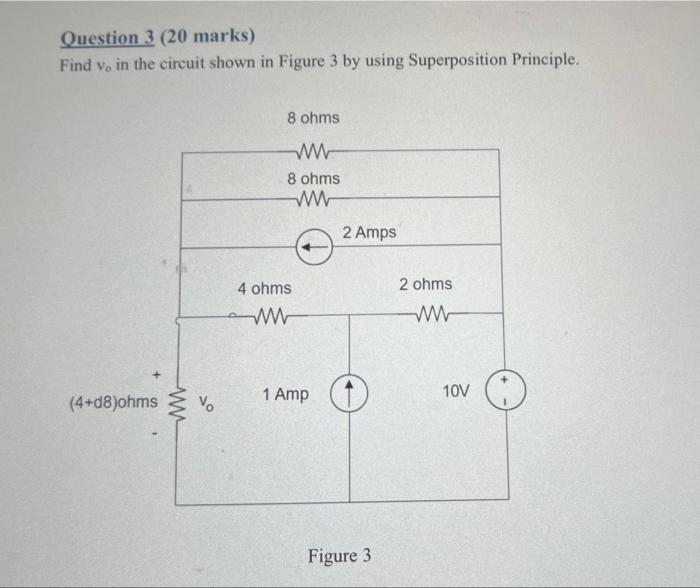# Question Question 3 (20 marks) Find $$v_{0}$$ in the circuit shown in Figure 3 by using Superposition Principle. Figure 3Transcribed Image Text: Question 3 (20 marks) Find $$v_{0}$$ in the circuit shown in Figure 3 by using Superposition Principle. Figure 3
Transcribed Image Text: Question 3 (20 marks) Find $$v_{0}$$ in the circuit shown in Figure 3 by using Superposition Principle. Figure 3&#12304;General guidance&#12305;The answer provided below has been developed in a clear step by step manner.Step1/1To apply the Superposition Principle, we need to analyze the circuit with only one source at a time and then combine the results.Step 1: Turn off the current source (set it to 0 A)To find the voltage v0 with the current source turned off, we can simplify the circuit as Since there is no current flowing through the 2-ohm resistor, we can remove it from the circuit. Also, we can combine the 8-ohm and 4-ohm resistors in parallel to get an equivalent resistance of 8/3 ohms. Now, we can use voltage division to find the voltage v0 across the (4 +d8) ohm resistor:\begin{align*} \mathrm{{v}{0}={10}{V}\times{\left[\frac{{{4}+{8}}}{{\frac{{8}}{{3}}+{4}+{8}}}\right]}={6.25}{V}} &= \end{align*}Step 2: Turn off the voltage source (set it to 0 V)To find the voltage v0 with the voltage source turned off, we can simplify the circuit Since there is no voltage across the 2-ohm resistor, we can remove it from the circuit. Also, we can combine the 8-ohm and 4-ohm resistors in parallel to get an equivalent resistance of 8 ... See the full answer